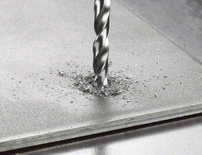Twist Drill Bits

HSS Twist Drill Bit Ground

HSS Twist Drill Bit offers solid drilling for standard usage with metal

New
HSS Twist Drill Bit Ground
HSS Twist Drill Bit offers solid drilling for standard usage with metalProduct highlights

HSS Twist Drill Bits Ground Sets

HSS Twist Drill Bit offers solid drilling for standard usage with metal

• 135-degree self-centring split-point tip combines active cutting and perfect centring without wandering
• Precision-ground bit made of HSS enables fast results
• No need to pre-punch or pilot drill up to 10 mm-diameter
Go to product range

Product descriptions

The HSS Twist Drill Bit Ground is for drilling through metal with maximum precision. The bit's exposed 135-degree self-centring split-point tip combines active cutting and perfect centring without wandering, delivering maximum precision. The split-point tip also eliminates any need to pre-punch or pilot drill for up to 10 mm. This precision-ground bit made of HSS (high-speed steel) enables up to 40% faster drilling rate and up to 50% lower feed pressure than standard-ground HSS drill bits with chisel edges. This bit is designed for drilling holes in alloyed and non-alloyed steel, cast steel, cast iron, sintered iron, malleable cast iron, non-ferrous metals and hard plastics. It has a cylindrical shank system (shank equal to drill bit diameter) and is intended for use in drill stands and drill drivers.

The HSS Twist Drill Bit Ground is manufactured to DIN 338. The drill bit is Type N (flute angle) with a 135-degree tip and a diameter tolerance of h8.

HSS Twist Drill Bit Ground
HSS Twist Drill Bit offers solid drilling for standard usage with metal135-degree self-centring split-point tip combines active cutting and perfect centring without wanderingPrecision-ground bit made of HSS enables fast resultsNo need to pre-punch or pilot drill up to 10 mm-diameter

Product range

4 Available product variations
Part number Diameter mm Working length mm Total length mm Set dimension(s) mm Pack quantity
2 607 019 114
2=0 / 3=0 / 4=0 / 5=0 / 6=0 - - - 5 Pcs
2 607 019 578
1=0 / 1=5 / 2=0 / 2=0 / 2=5 / 3=0 / 3=0 / 3=5 / 4=0 / 4=0 / 4=5 / 5=0 / 5=5 / 6=0 / 7=0 / 8=0 / 9=0 / 10=0 12 / 18 / 24 / 24 / 30 / 33 / 33 / 39 / 43 / 43 / 47 / 52 / 57 / 57 / 69 / 75 / 81 / 87 34 / 40 / 49 / 49 / 57 / 61 / 61 / 70 / 75 / 75 / 80 / 86 / 93 / 93 / 109 / 117 / 125 / 133 180=0 x 85=0 x 45 18 Pcs
2 608 587 013
1=0 / 1=5 / 2=0 / 2=5 / 3=0 / 3=5 / 4=0 / 4=5 / 5=0 / 5=5 / 6=0 / 6=5 / 7=0 / 7=5 / 8=0 / 8=5 / 9=0 / 9=5 / 10=0 12 / 18 / 24 / 30 / 33 / 39 / 43 / 47 / 52 / 57 / 57 / 63 / 69 / 69 / 75 / 75 / 81 / 81 / 87 - 172=0 x 115=0 x 42 19 Pcs
2 608 587 017
1=0 / 1=5 / 2=0 / 2=5 / 3=0 / 3=5 / 4=0 / 4=5 / 5=0 / 5=5 / 6=0 / 6=5 / 7=0 / 7=5 / 8=0 / 8=5 / 9=0 / 9=5 / 10=0 / 10=5 / 11=0 / 11=5 / 12=0 / 12=5 / 13=0 12 / 18 / 24 / 30 / 33 / 39 / 43 / 47 / 52 / 57 / 57 / 63 / 69 / 69 / 75 / 75 / 81 / 81 / 87 / 87 / 94 / 94 / 101 / 101 / 101 - 200=0 x 115=0 x 58 25 Pcs

Similar accessories

Check out similar accessories
Service Hotline

Have any questions about our repair and spare parts service?